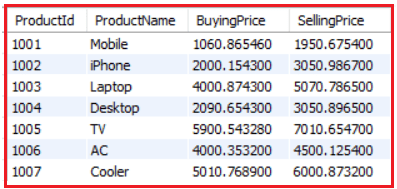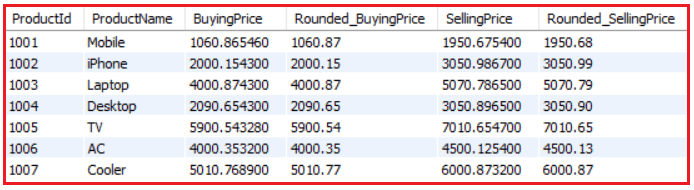# MySQL Round Function

## MySQL Round Function with Examples

In this article, I am going to discuss MySQL Round Function with Examples. Please read our previous article where we discussed MySQL LENGTH and CHAR_LENGTH Function with Examples.

##### MySQL Round Function

The ROUND function is used to round a floating-point number to given decimal numbers. The ROUND function only works on numeric columns. In simple words, we can say that the MySQL ROUND function is a mathematical function that allows us to round a number to a certain number of decimal places.

##### MySQL ROUND Function Syntax:

Following is the syntax to use the Round function.

ROUND(number, [decimals])

###### Parameters:

The MySQL ROUND function accepts two parameters as follows:

1. number: Required. The number to be rounded
2. decimals: Optional. It defaults to zero if you skip it. The Number of decimal places up to which the given number is to be rounded. If not given it round off the number to the closest integer (no decimals). If it is negative, then the number is rounded to the left side of the decimal point.

Returns: It returns the number after rounded to the specified places.

Note: If decimals are a negative number, then the ROUND function will make digits to the left of the decimal place 0 values.

###### Example: Rounding off a number when Decimal is not specified.

Positive Number:
SELECT ROUND(50.55) AS Round_Number;
Output: 50

Negative Number:
SELECT ROUND(-15.25) AS Round_Number;
Output: -15

###### Example: Rounding off a number when the decimal is positive.

Rounding a Positive number up to 3 decimal places
SELECT ROUND(22516.67519, 3) AS Round_Number;
Output: 22516.675

Rounding a Negative number up to 3 decimal places
SELECT ROUND(-22516.67519, 3) AS Round_Number;
Output: -22516.675

###### Example: Rounding off a number when the decimal is negative.

Rounding a Positive number up to 2 decimal places
SELECT ROUND(22516.67519, -2) AS Round_Number;
Output: 22500

Rounding a Negative number up to 3 decimal places
SELECT ROUND(-22516.67519, -3) AS Round_Number;
Output: -22500

###### Example: Rounding off a number to 0 decimal places.

Rounding a Positive number up to 0 decimal places
SELECT ROUND(123.456, 0);
Output: 123

Rounding a Negative number up to 0 decimal places
SELECT ROUND(-123.456, 0);
Output: -123

##### Working with database table:

The MySQL ROUND Function can also be used to find the rounded values for the database table column data. To understand this concept, we are going to use the following Product table.Please use the below SQL Script to create and populate the Product table with the required sample data.

```CREATE TABLE Product(
ProductId INT PRIMARY KEY,
ProductName VARCHAR(100) NOT NULL,
SellingPrice DECIMAL(13, 6) NOT NULL
);

INSERT INTO Product VALUES (1001, 'Mobile', 1060.865460, 1950.675400);
INSERT INTO Product VALUES (1002, 'iPhone', 2000.154300, 3050.986700);
INSERT INTO Product VALUES (1003, 'Laptop', 4000.874300, 5070.786500);
INSERT INTO Product VALUES (1004, 'Desktop', 2090.654300, 3050.896500);
INSERT INTO Product VALUES (1005, 'TV', 5900.543280, 7010.654700);
INSERT INTO Product VALUES (1006, 'AC', 4000.353200, 4500.125400);
INSERT INTO Product VALUES (1007, 'Cooler', 5010.768900, 6000.873200);
```

Now, we are going to find the rounded values for the Price column of the Product table by executing the below SQL script.

```SELECT ProductId, ProductName,